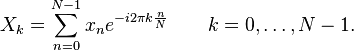MATLAB : Fast Fourier Transform (FFT) & Inverse Fast Fourier Transform (IFFT) , Without Using built in MATLAB function

MATLAB based Fast Fourier Transform (FFT) & Inverse Fast Fourier Transform (IFFT).
The following MATLAB implemented method of finding the FFT is based on the formula given below:

X(k)= Σ x(n)e^(-j*2*pi*k*n/N)
Σ is from k=0 to N-1$X_k = \sum_{n=0}^{N-1} x_n e^{-{i 2\pi k \frac{n}{N}}}\qquadk = 0,\dots,N-1. MATLAB based Fast Fourier Transform (FFT) & Inverse Fast Fourier Transform (IFFT) Calculation, Without Using fft & ifft inbuilt MATLAB function$

Similarly we have used the formula for IFFT as:

x(n)= (1/N)[Σ X(K)e^(j*2*pi*k*n/N)]
Σ is from n=0 to N-1

If you want to make your work for calculating the FFT & IFFT function even more simpler, then you can feed in your sequence directly to fft() function of MATLAB.

MATLAB Code for calculating FFT (Fast Fourier Transform) & IFFT (Inverse Fast Fourier Transform):

clc;
close all;
clear all;
x = input('Enter the sequence for FFT = ');
N = length(x);
%finding the length of entered sequence for N point FFT

for k = 1:N %for every value of k
y(k) = 0;
for n = 1:N
%for every value of n
y(k) = y(k)+x(n)*exp(-1i*2*pi*(k-1)*(n-1)/N);
%as per FFT formula 0 to n-1
end
end

t = 0:N-1;
subplot(3,1,1);
stem(t,x);
% for discrete plotting
%plotting input sequence
ylabel('Amplitude');
xlabel('n');
title('Input');

magnitude = abs(y);
% absolute values of individual FFT complex
disp('FFT : ');
disp(magnitude);

t = 0:N-1;
subplot(3,1,2);
stem(t,magnitude);

%plotting FFT sequence
ylabel('Amplitude');
xlabel('K');
title('FFT');

R = length(y);
%length of the fft array

for n = 1:R
x1(n) = 0;
for k = 1:R
%loop as per the IFFT formula
x1(n) = x1(n)+(1/R)*y(k)*exp(1i*2*pi*(k-1)*(n-1)/R);
end
end

t = 0:R-1;
subplot(3,1,3);
stem(t,x1);

%ploting IFFT sequence
disp('IFFT :');
disp(x1);
ylabel('Amplitude');
xlabel('n');
title('IFFT');

Sample Input & Output:

Enter the sequence for FFT = [1 2 3 4 5]

FFT Sequence =
15.0000    4.2533    2.6287    2.6287    4.2533

IFFT Sequence =

1.0000 - 0.0000i    2.0000 - 0.0000i    3.0000    4.0000   5.0000 + 0.0000i

Output Graph:MATLAB based Fast Fourier Transform (FFT) & Inverse Fast Fourier Transform (IFFT) Calculation, Without Using fft & ifft inbuilt MATLAB function

Read More At Digital iVision Labs:

#FSK Simulation Code Using MATLAB
#Phase Shift Keying (PSK) Modulation MATLAB Simulation, With MATLAB Code
#Amplitude Shift Keying (ASK) Modulation MATLAB Simulation, With MATLAB Code

#Matlab Christmas Tree Plot- Christmas celebration through matlab way - Merry Christmas!

1 comment:

1.Sir, I am final year student of m.tech ,I am doing project on "dvb-t2 technology used in mobile reception to reduce the battery power ",so please send me the code please help me